# 17. Training a Neural Network with Python

## Introduction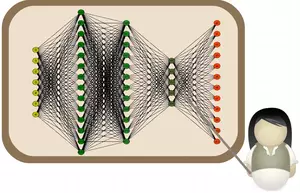In the chapter Running Neural Networks, we programmed a class in Python code called 'NeuralNetwork'. The instances of this class are networks with three layers. When we instantiate an ANN of this class, the weight matrices between the layers are automatically and randomly chosen. It is even possible to run such a ANN on some input, but naturally it doesn't make a lot of sense exept for testing purposes. Such an ANN cannot provide correct classification results. In fact, the classification results are in no way adapted to the expected results. The values of the weight matrices have to be set according the the classification task. We need to improve the weight values, which means that we have to train our network. To train it we have to implement backpropagation in the train method. If you don't understand backpropagation and want to understand it, we recommend to go back to the chapter Backpropagation in Neural Networks.

After knowing und hopefully understanding backpropagation, you are ready to fully understand the train method.

The train method is called with an input vector and a target vector. The shape of the vectors can be one-dimensional, but they will be automatically turned into the correct two-dimensional shape, i.e. reshape(input_vector.size, 1) and reshape(target_vector.size, 1). After this we call the run method to get the result of the network output_vector_network = self.run(input_vector). This output may differ from the target_vector. We calculate the output_error by subtracting the output of the network output_vector_network from the target_vector.

%%writefile neural_networks1.py
import numpy as np
from scipy.special import expit as activation_function
from scipy.stats import truncnorm

def truncated_normal(mean=0, sd=1, low=0, upp=10):
return truncnorm(
(low - mean) / sd, (upp - mean) / sd, loc=mean, scale=sd)

class NeuralNetwork:

def __init__(self,
no_of_in_nodes,
no_of_out_nodes,
no_of_hidden_nodes,
learning_rate):
self.no_of_in_nodes = no_of_in_nodes
self.no_of_out_nodes = no_of_out_nodes
self.no_of_hidden_nodes = no_of_hidden_nodes
self.learning_rate = learning_rate
self.create_weight_matrices()

def create_weight_matrices(self):
""" A method to initialize the weight matrices of the neural network"""
self.weights_in_hidden = X.rvs((self.no_of_hidden_nodes,
self.no_of_in_nodes))
self.weights_hidden_out = X.rvs((self.no_of_out_nodes,
self.no_of_hidden_nodes))

def train(self, input_vector, target_vector):
"""
input_vector and target_vector can be tuples, lists or ndarrays
"""
# make sure that the vectors have the right shape
input_vector = np.array(input_vector)
input_vector = input_vector.reshape(input_vector.size, 1)
target_vector = np.array(target_vector).reshape(target_vector.size, 1)

output_vector_hidden = activation_function(self.weights_in_hidden @ input_vector)
output_vector_network = activation_function(self.weights_hidden_out @ output_vector_hidden)

output_error = target_vector - output_vector_network
tmp = output_error * output_vector_network * (1.0 - output_vector_network)
self.weights_hidden_out += self.learning_rate  * (tmp @ output_vector_hidden.T)

# calculate hidden errors:
hidden_errors = self.weights_hidden_out.T @ output_error
# update the weights:
tmp = hidden_errors * output_vector_hidden * (1.0 - output_vector_hidden)
self.weights_in_hidden += self.learning_rate * (tmp @ input_vector.T)

def run(self, input_vector):
"""
running the network with an input vector 'input_vector'.
'input_vector' can be tuple, list or ndarray
"""
# make sure that input_vector is a column vector:
input_vector = np.array(input_vector)
input_vector = input_vector.reshape(input_vector.size, 1)
input4hidden = activation_function(self.weights_in_hidden @ input_vector)
output_vector_network = activation_function(self.weights_hidden_out @ input4hidden)
return output_vector_network

def evaluate(self, data, labels):
"""
Counts how often the actual result corresponds to the
target result.
A result is considered to be correct, if the index of
the maximal value corresponds to the index with the "1"
in the one-hot representation,
e.g.
res = [0.1, 0.132, 0.875]
labels[i] = [0, 0, 1]
"""
corrects, wrongs = 0, 0
for i in range(len(data)):
res = self.run(data[i])
res_max = res.argmax()
if res_max == labels[i].argmax():
corrects += 1
else:
wrongs += 1
return corrects, wrongs


### OUTPUT:

Writing neural_networks1.py


We assume that you save the previous code in a file called neural_networks1.py. We will use it under this name in the coming examples.

To test this neural network class we need train and test data. We create the data with make_blobs from sklearn.datasets.

from sklearn.datasets import make_blobs

n_samples = 500
blob_centers = ([2, 6], [6, 2], [7, 7])
n_classes = len(blob_centers)
data, labels = make_blobs(n_samples=n_samples,
centers=blob_centers,
random_state=7)


Let us visualize the previously created data:

import matplotlib.pyplot as plt

colours = ('green', 'red', "yellow")
fig, ax = plt.subplots()

for n_class in range(n_classes):
ax.scatter(data[labels==n_class][:, 0],
data[labels==n_class][:, 1],
c=colours[n_class],
s=40,
label=str(n_class))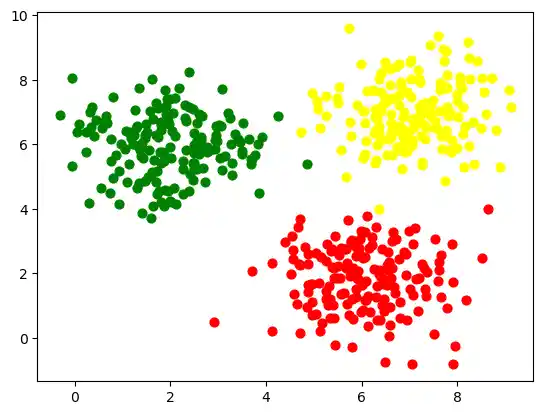The labels are wrongly represented. They are in a one-dimensional vector:

labels[:7]


### OUTPUT:

array([2, 2, 1, 0, 2, 0, 1])


We need a one-hot representation for each label. So the labels are represented as

Label One-Hot Representation
0 (1, 0, 0)
1 (0, 1, 0)
2 (0, 0, 1)

We can easily change the labels with the following commands:

import numpy as np

labels = np.arange(n_classes) == labels.reshape(labels.size, 1)
labels = labels.astype(np.float64)
labels[:7]


### OUTPUT:

array([[0., 0., 1.],
[0., 0., 1.],
[0., 1., 0.],
[1., 0., 0.],
[0., 0., 1.],
[1., 0., 0.],
[0., 1., 0.]])


We are ready now to create a train and a test data set:

from sklearn.model_selection import train_test_split

res = train_test_split(data, labels,
train_size=0.8,
test_size=0.2,
random_state=42)
train_data, test_data, train_labels, test_labels = res
train_labels[:10]


### OUTPUT:

array([[0., 0., 1.],
[0., 1., 0.],
[1., 0., 0.],
[0., 0., 1.],
[0., 0., 1.],
[1., 0., 0.],
[0., 1., 0.],
[1., 0., 0.],
[1., 0., 0.],
[0., 0., 1.]])


We create a neural network with two input nodes, and three output nodes. One output node for each class:

from neural_networks1 import NeuralNetwork

simple_network = NeuralNetwork(no_of_in_nodes=2,
no_of_out_nodes=3,
no_of_hidden_nodes=5,
learning_rate=0.3)


The next step consists in training our network with the data and labels from our training samples:

for i in range(len(train_data)):
simple_network.train(train_data[i], train_labels[i])


We now have to check how well our network has learned. For this purpose, we will use the evaluate function:

simple_network.evaluate(train_data, train_labels)


### OUTPUT:

(392, 8)


Live Python training

Enjoying this page? We offer live Python training courses covering the content of this site.

Enrol here

## Neural Network with Bias Nodes

We already introduced the basic idea and necessity of bias nodes in the chapter "Simple Neural Network", in which we focussed on very simple linearly separable data sets. We learned that a bias node is a node that is always returning the same output. In other words: It is a node which is not depending on some input and it does not have any input. The value of a bias node is often set to one, but it can be set to other values as well. Except for zero, which makes no sense for obvious reasons. If a neural network does not have a bias node in a given layer, it will not be able to produce output in the next layer that differs from 0 when the feature values are 0. Generally speaking, we can say that bias nodes are used to increase the flexibility of the network to fit the data. Usually, there will be not more than one bias node per layer. The only exception is the output layer, because it makes no sense to add a bias node to this layer.

The following diagram shows the first two layers of our previously used three-layered neural network: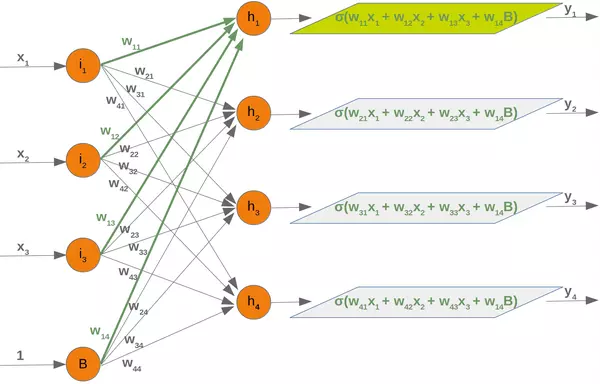We can see from this diagram that our weight matrix needs one additional column and the bias value has to be added to the input vector: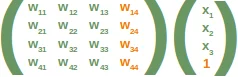Again, the situation for the weight matrix between the hidden and the output layer is similar: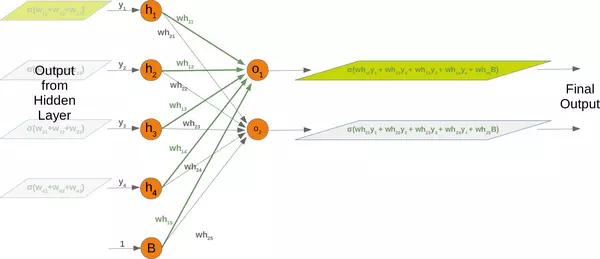The same is true for the corresponding matrix: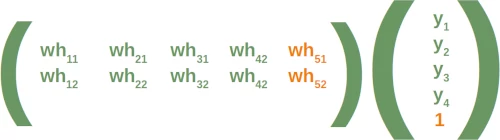The following is a complete Python class implementing our network with bias nodes:

%%capture
%%writefile neural_networks2.py

import numpy as np
from scipy.stats import truncnorm
from scipy.special import expit as activation_function

def truncated_normal(mean=0, sd=1, low=0, upp=10):
return truncnorm(
(low - mean) / sd, (upp - mean) / sd, loc=mean, scale=sd)

class NeuralNetwork:

def __init__(self,
no_of_in_nodes,
no_of_out_nodes,
no_of_hidden_nodes,
learning_rate,
bias=None):
self.no_of_in_nodes = no_of_in_nodes
self.no_of_hidden_nodes = no_of_hidden_nodes
self.no_of_out_nodes = no_of_out_nodes
self.learning_rate = learning_rate
self.bias = bias
self.create_weight_matrices()

def create_weight_matrices(self):
""" A method to initialize the weight matrices of the neural
network with optional bias nodes"""
bias_node = 1 if self.bias else 0
rad = 1 / np.sqrt(self.no_of_in_nodes + bias_node)
self.weights_in_hidden = X.rvs((self.no_of_hidden_nodes,
self.no_of_in_nodes + bias_node))
rad = 1 / np.sqrt(self.no_of_hidden_nodes + bias_node)
self.weights_hidden_out = X.rvs((self.no_of_out_nodes,
self.no_of_hidden_nodes + bias_node))

def train(self, input_vector, target_vector):
""" input_vector and target_vector can be tuple, list or ndarray """

# make sure that the vectors have the right shap
input_vector = np.array(input_vector)
input_vector = input_vector.reshape(input_vector.size, 1)
if self.bias:
# adding bias node to the end of the input_vector
input_vector = np.concatenate( (input_vector, [[self.bias]]) )
target_vector = np.array(target_vector).reshape(target_vector.size, 1)

output_vector_hidden = activation_function(self.weights_in_hidden @ input_vector)
if self.bias:
output_vector_hidden = np.concatenate( (output_vector_hidden, [[self.bias]]) )
output_vector_network = activation_function(self.weights_hidden_out @ output_vector_hidden)

output_error = target_vector - output_vector_network
# update the weights:
tmp = output_error * output_vector_network * (1.0 - output_vector_network)
self.weights_hidden_out += self.learning_rate  * (tmp @ output_vector_hidden.T)

# calculate hidden errors:
hidden_errors = self.weights_hidden_out.T @ output_error
# update the weights:
tmp = hidden_errors * output_vector_hidden * (1.0 - output_vector_hidden)
if self.bias:
x = (tmp @input_vector.T)[:-1,:]     # last row cut off,
else:
x = tmp @ input_vector.T
self.weights_in_hidden += self.learning_rate *  x

def run(self, input_vector):
"""
running the network with an input vector 'input_vector'.
'input_vector' can be tuple, list or ndarray
"""
# make sure that input_vector is a column vector:
input_vector = np.array(input_vector)
input_vector = input_vector.reshape(input_vector.size, 1)
if self.bias:
# adding bias node to the end of the inpuy_vector
input_vector = np.concatenate( (input_vector, []) )
input4hidden = activation_function(self.weights_in_hidden @ input_vector)
if self.bias:
input4hidden = np.concatenate( (input4hidden, []) )
output_vector_network = activation_function(self.weights_hidden_out @ input4hidden)
return output_vector_network

def evaluate(self, data, labels):
corrects, wrongs = 0, 0
for i in range(len(data)):
res = self.run(data[i])
res_max = res.argmax()
if res_max == labels[i].argmax():
corrects += 1
else:
wrongs += 1
return corrects, wrongs


We can use again our previously created classes to test our classifier:

from neural_networks2 import NeuralNetwork

simple_network = NeuralNetwork(no_of_in_nodes=2,
no_of_out_nodes=3,
no_of_hidden_nodes=5,
learning_rate=0.1,
bias=1)

for i in range(len(train_data)):
simple_network.train(train_data[i], train_labels[i])

simple_network.evaluate(train_data, train_labels)


### OUTPUT:

(394, 6)


## Exercise

We created in the chapter "Data Creation" a file strange_flowers.txt in the folder data. Create a Neural Network to classify the 'flowers':

The data looks like this:

0.000,240.000,100.000,3.020
253.000,99.000,13.000,3.875
202.000,107.000,6.000,4.1
186.000,84.000,6.000,4.068
0.000,244.000,103.000,3.386
0.000,246.000,98.000,2.955
241.000,103.000,3.000,4.049
236.000,104.000,12.000,3.087
244.000,109.000,1.000,3.111
253.000,97.000,8.000,3.752
231.000,92.000,1.000,3.488
0.000,250.000,103.000,3.379


Live Python training

Enjoying this page? We offer live Python training courses covering the content of this site.

Upcoming online Courses

Enrol here

## Solution:

import numpy as np

data = c[:, :-1]
labels = c[:, -1]
n_classes = int(np.max(labels)) # in our case 1, ... 4
data[:5]


### OUTPUT:

array([[238.  , 106.  ,  16.  ,   4.19],
[246.  , 120.  ,  13.  ,   3.78],
[239.  , 103.  ,   0.  ,   4.03],
[248.  , 110.  ,   2.  ,   3.75],
[242.  , 122.  ,  15.  ,   4.01]])

labels[:10]


### OUTPUT:

array([1., 1., 1., 1., 1., 1., 1., 1., 1., 1.])

# turning labels into one-hot representation:
labels_one_hot = np.arange(1, n_classes+1) == labels.reshape(labels.size, 1)
labels_one_hot = labels_one_hot.astype(np.float64)
labels_one_hot[:3]


### OUTPUT:

array([[1., 0., 0., 0.],
[1., 0., 0., 0.],
[1., 0., 0., 0.]])


We need to scale our data, because unscaled input data can result in a slow or unstable learning process. We will use the function scale from sklearn/preprocessing . It standardizes a dataset along any axis. It centers to the mean and component wise scale to unit variance.

from sklearn.model_selection import train_test_split

res = train_test_split(data, labels_one_hot,
train_size=0.8,
test_size=0.2,
random_state=42)
train_data, test_data, train_labels, test_labels = res
train_labels[:10]


### OUTPUT:

array([[0., 1., 0., 0.],
[0., 1., 0., 0.],
[0., 0., 1., 0.],
[0., 1., 0., 0.],
[0., 0., 1., 0.],
[0., 1., 0., 0.],
[1., 0., 0., 0.],
[1., 0., 0., 0.],
[0., 0., 1., 0.],
[0., 1., 0., 0.]])

from sklearn.preprocessing import StandardScaler
scaler = StandardScaler()
train_data = scaler.fit_transform(train_data) #  fit and transform
test_data = scaler.transform(test_data) #  transform

from neural_networks2 import NeuralNetwork

simple_network = NeuralNetwork(no_of_in_nodes=4,
no_of_out_nodes=4,
no_of_hidden_nodes=20,
learning_rate=0.3)

for i in range(len(train_data)):
simple_network.train(train_data[i], train_labels[i])

simple_network.evaluate(train_data, train_labels)


### OUTPUT:

(615, 21)


Live Python training

Enjoying this page? We offer live Python training courses covering the content of this site.

Upcoming online Courses

Enrol here